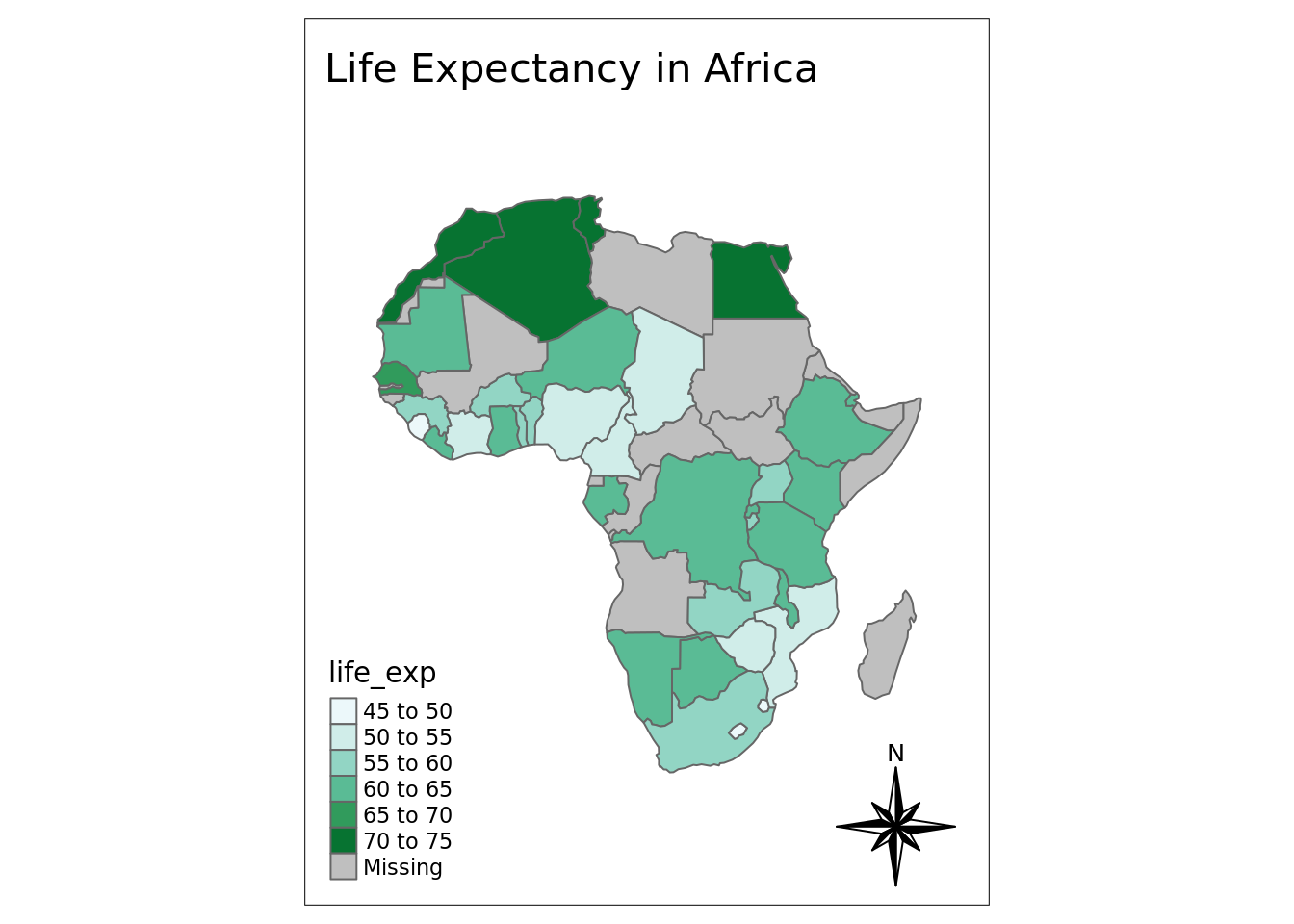# 3 Simple Static Maps

R can be used for spatial visualization and analysis, with features that rival commercial GIS software. There are many R packages to perform spatial analysis, and advanced spatial analysis is its own subject, beyond the scope of this class. Here, I will focus on relatively simple static maps. We will use several spatial packages in conjunction with the venerable `ggplot2`, a general purpose package for plotting.

## 3.1 Setup

We must load several packages to get started. We will also set `ggplot2` to display in `theme_bw` which will make our plots clear and simple by default.

``````library(sf) #'simple features' package
library(leaflet) # web-embeddable interactive maps
library(ggplot2) # general purpose plotting
library(rnaturalearth) # map data
library(rnaturalearthdata)# map data
library(ggspatial) # scale bars and north arrows

#the package below was installed from source through github:
# devtools::install_github("ropensci/rnaturalearthhires"))
library(rnaturalearthhires)# map data

theme_set(theme_bw())``````

## 3.2 Data

Spatial data come in many forms. Here, we are going to make use of spatial data that comes pre-loaded in the package `rnaturalearth`. Below we select medium-resolution map data for the entire world and higher resolution data for Tanzania in particular.

``````world <- ne_countries(scale="medium", returnclass = "sf")
tanzania <- ne_countries(country="united republic of tanzania", type="countries", scale='large', returnclass = "sf")``````

## 3.3 A map of the world

Here we create a basic map of the world. This call to `ggplot()` should look similar to previous plotting code that you have seen and used with this package. The spatial plotting occurs via `geom_sf()` which is made available through the `sf` package. Also please note that layers are added one at a time in a `ggplot` call, so the order of each layer is important.

``````ggplot(data = world) +
geom_sf()``````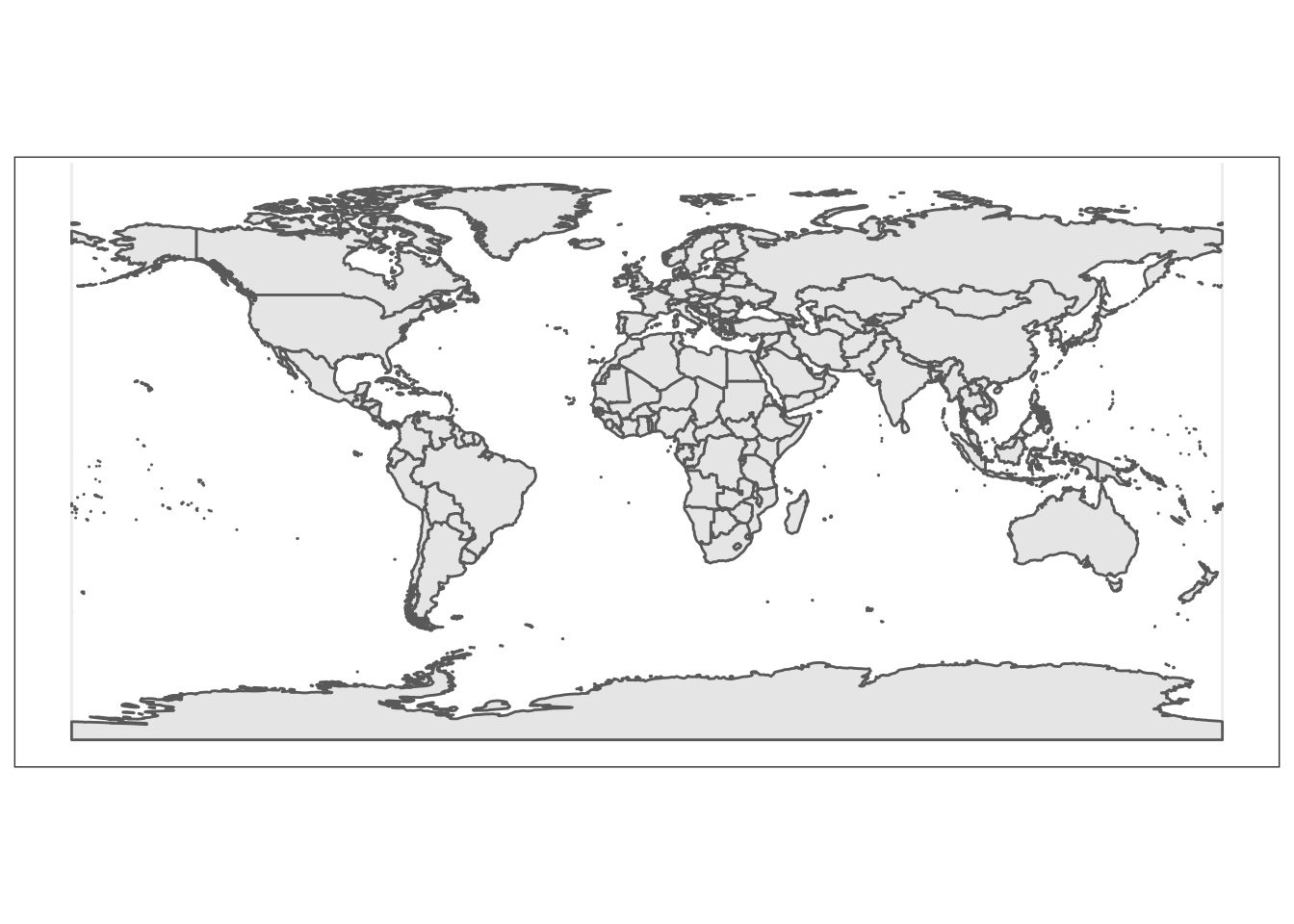## 3.4 A map of Tanzania

Here we create a basic map of one nation, Tanzania.

``````ggplot(data = tanzania) +
geom_sf()``````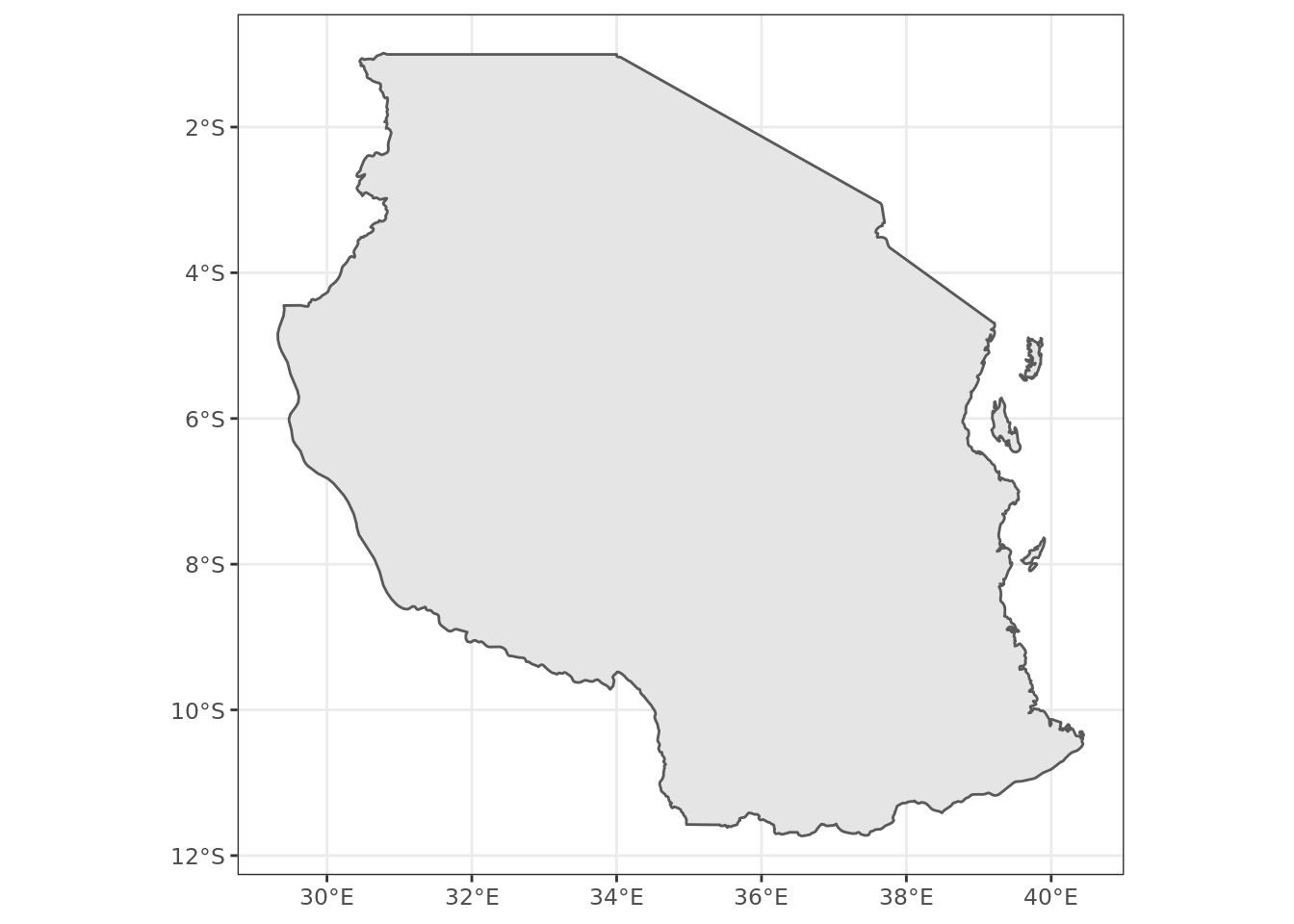## 3.5 Adding titles and axis labels

A title and a subtitle can be added to the map using the function `ggtitle`. Axis names can be added using `xlab` and `ylab`

``````ggplot(data = tanzania) +
geom_sf() +
xlab("Longitude") + ylab("Latitude") +
ggtitle("The United Republic of Tanzania")``````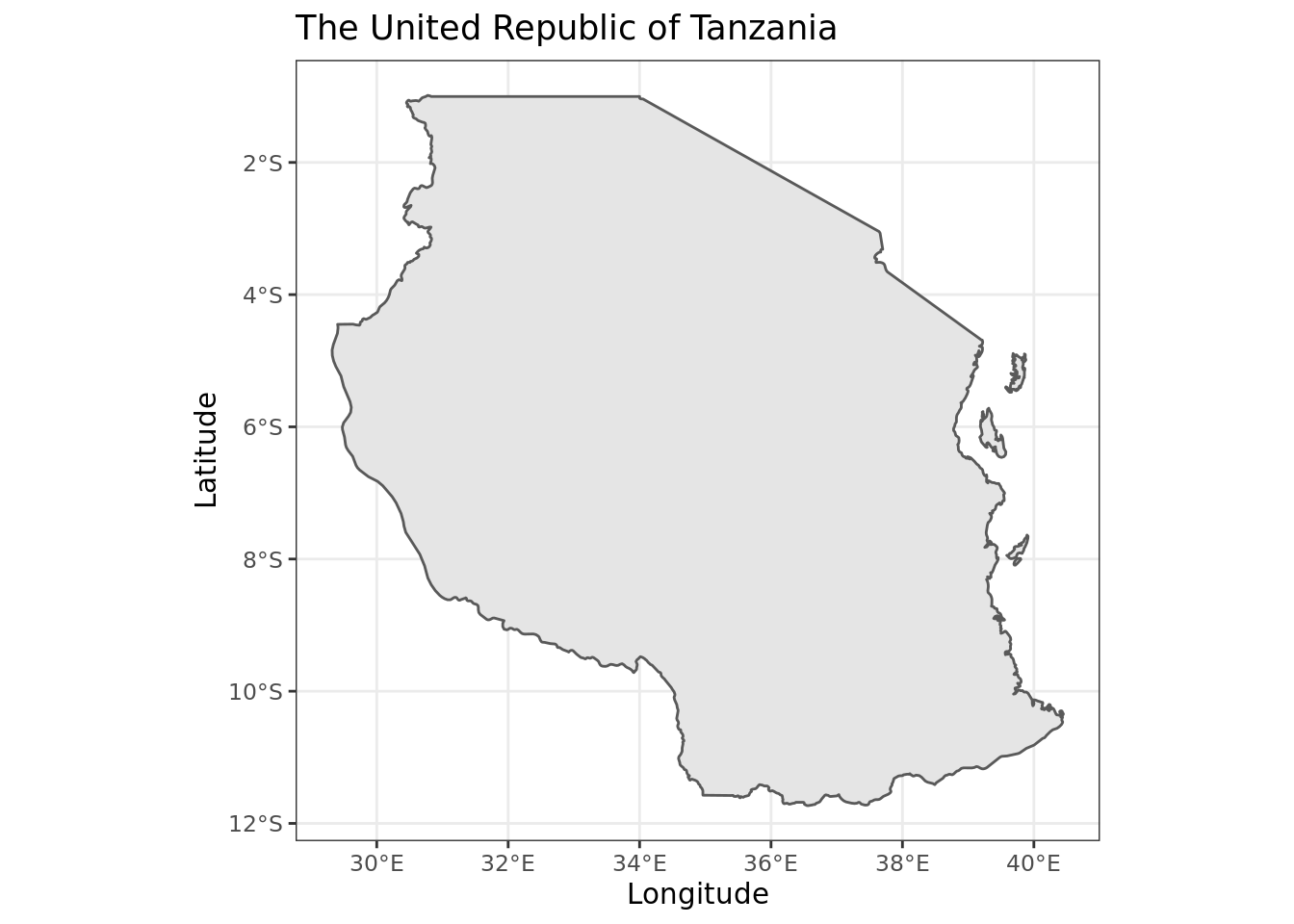## 3.6 Adding scale bars and a north arrow

We use here the package `ggspatial`, which provides an easy function for creating scale bars / north arrows.

``````ggplot(data = tanzania) +
geom_sf() +
xlab("Longitude") + ylab("Latitude") +
ggtitle("The United Republic of Tanzania") +
annotation_scale(location = "bl", width_hint = 0.4) +
annotation_north_arrow(location = "bl", which_north = "true",
style = north_arrow_fancy_orienteering)``````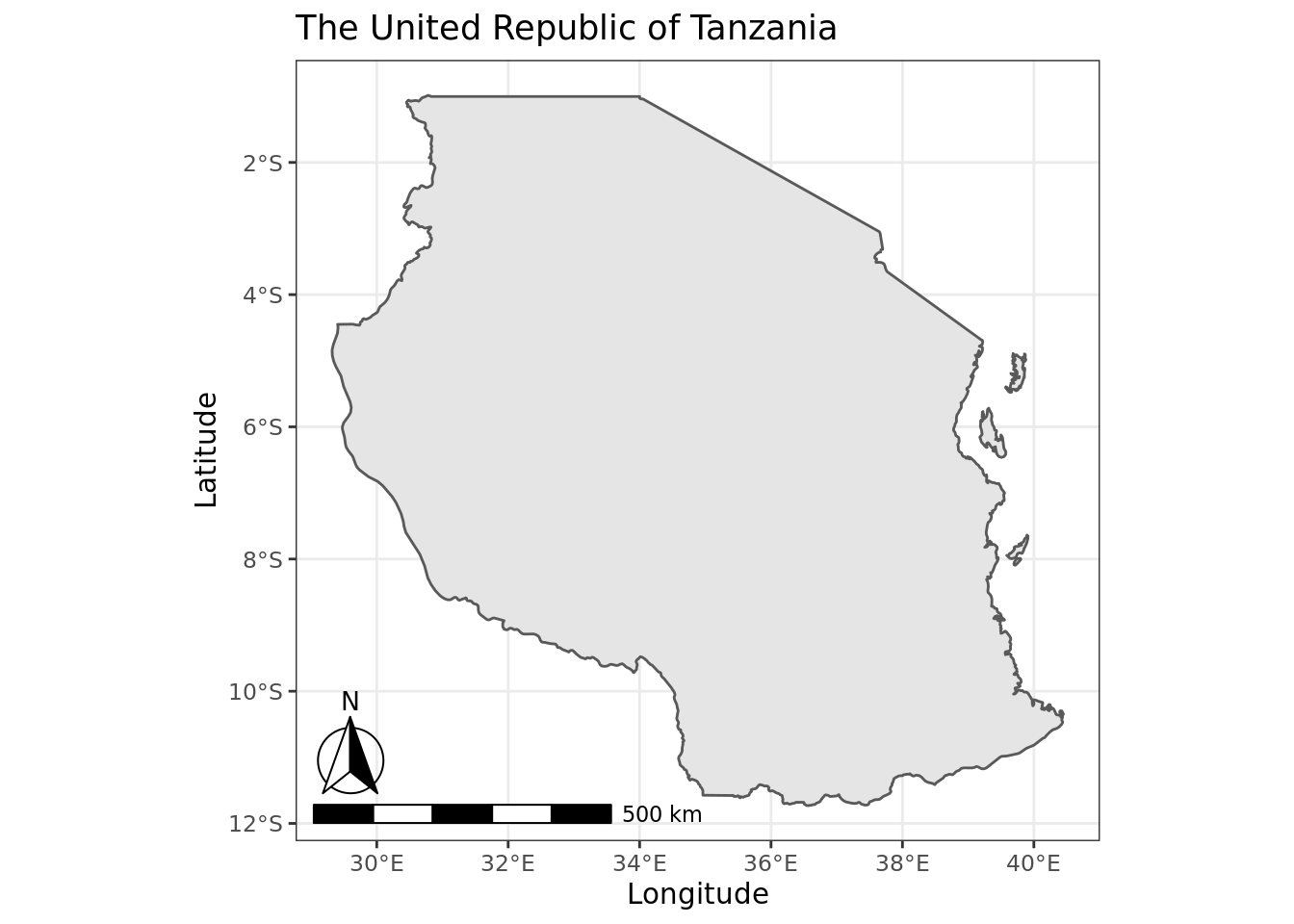In the example above, we used functions in `ggspatial` to add a north symbol and a scale bar into the map. Explanations of the arguments that we have set manually include:

• `location = "bl"`: where to put the scale bar (“tl” for top left, etc.).
• `width_hint = 0.4`: The (suggested) proportion of the plot area which the scalebar should occupy.
• `which_north = "true"`: “true” always points to the north pole from whichever corner of the map the north arrow is in. One can also specify “grid” which results in a north arrow always pointing up;
• `pad_x = unit(0.0, "in")`: Distance between scale bar and x-axis (longitude) edge of panel, in inches
• `pad_y = unit(0.2, "in")`: Distance between scale bar and y-axis (latitude) edge of panel, in inches
• `style = north_arrow_fancy_orienteering` what style of arrow to show. Other options include `north_arrow_orienteering`, `north_arrow_minimal`.

## 3.7 Further tweaking of the scale bars and north arrow

It is possible to change the font size for the legend of the scale bar (argument `legend_size`, which defaults to 3). The North arrow behind the “N” north symbol can also be adjusted for its length (`arrow_length`), its distance to the scale (`arrow_distance`), or the size the N north symbol itself (`arrow_north_size`, which defaults to 6).

## 3.8 Adding points to the map

The top five most populous cities in Tanzania happen to be: Dar es salaam, Mwanza, Arusha, Dodoma, and Mbeya. I’d like to add points and text labels to our map to represent those cities. We do this by creating a new dataframe, `city_data` and then adding those data to the map using the functions `geom_point` and `geom_text`.

``````
city_data <- data.frame(city_name=c("Dar es Salaam", "Mwanza", "Arusha", "Dodoma", "Mbeya"))
city_data\$lat <- c(-6.7924,-2.5164,-3.3869,-6.1630,-8.9094)
city_data\$lon <- c(39.2083,32.9175,36.6830,35.7516,33.4608)

ggplot(data = tanzania) +
geom_sf() +
xlab("Longitude") + ylab("Latitude") +
ggtitle("Major Cities in the United Republic of Tanzania") +
annotation_scale(location = "bl", width_hint = 0.4) +
annotation_north_arrow(location = "bl", which_north = "true",
style = north_arrow_fancy_orienteering) +
geom_point(data = city_data, mapping = aes(x = lon, y = lat), colour = "red") +
geom_text(data = city_data, mapping=aes(x=lon, y=lat, label=city_name), nudge_y = 0.5, color="darkblue")``````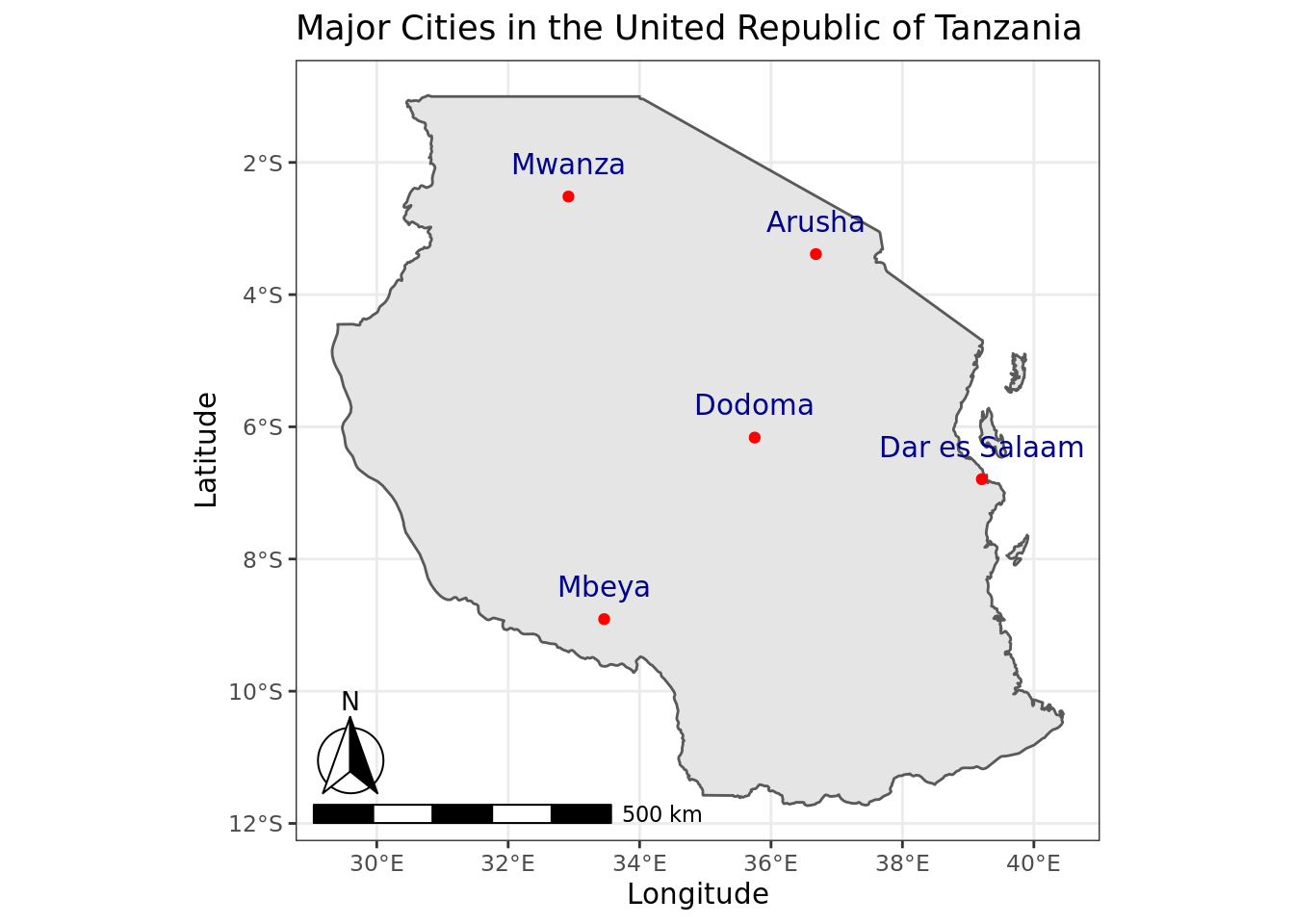Explanations of the function arguments include:

• `data = city_data` this provides the new data to be used in the new point and textlabel layers to the map.
• `mapping = aes(x = lon, y = lat)` this specifies exactly how the data in `city_data` correspond to the necessary aesthetic (or `aes`) elements of any `geom_point`, which include the x coordinates ( corresponding to our column `lon`) and the y coordinates (corresponding to `lat`).
• `colour = "red"` the color to use for the points. A reference to different color names that can be used in ggplot2 is available here.
• `label=city_name` geom_text elements require a specification for the `label` aesthetic – this is the text to be displayed.
• `nudge_y = 0.5` this sets how the label should be displayed, relative to its x and y coordinates. In this case, we are just pushing the label a bit higher in the y-axis, (moving it north) so that it does not display directly on top of the point displayed for each city.

## 3.9 Zoom and Pan your map

The frame of view of your map can be set using `coord_sf` function.

``````
ggplot(data = tanzania) +
geom_sf() +
coord_sf(xlim = c(35.5, 38), ylim = c(-4,-2), expand = FALSE) +
xlab("Longitude") + ylab("Latitude") +
ggtitle("Arusha") +
annotation_scale(location = "bl", width_hint = 0.4) +
annotation_north_arrow(location = "bl", which_north = "true",
style = north_arrow_fancy_orienteering) +
geom_point(data = city_data, mapping = aes(x = lon, y = lat), colour = "red") +
geom_text(data = city_data, mapping=aes(x=lon, y=lat, label=city_name), nudge_y = 0.1, color="darkblue")``````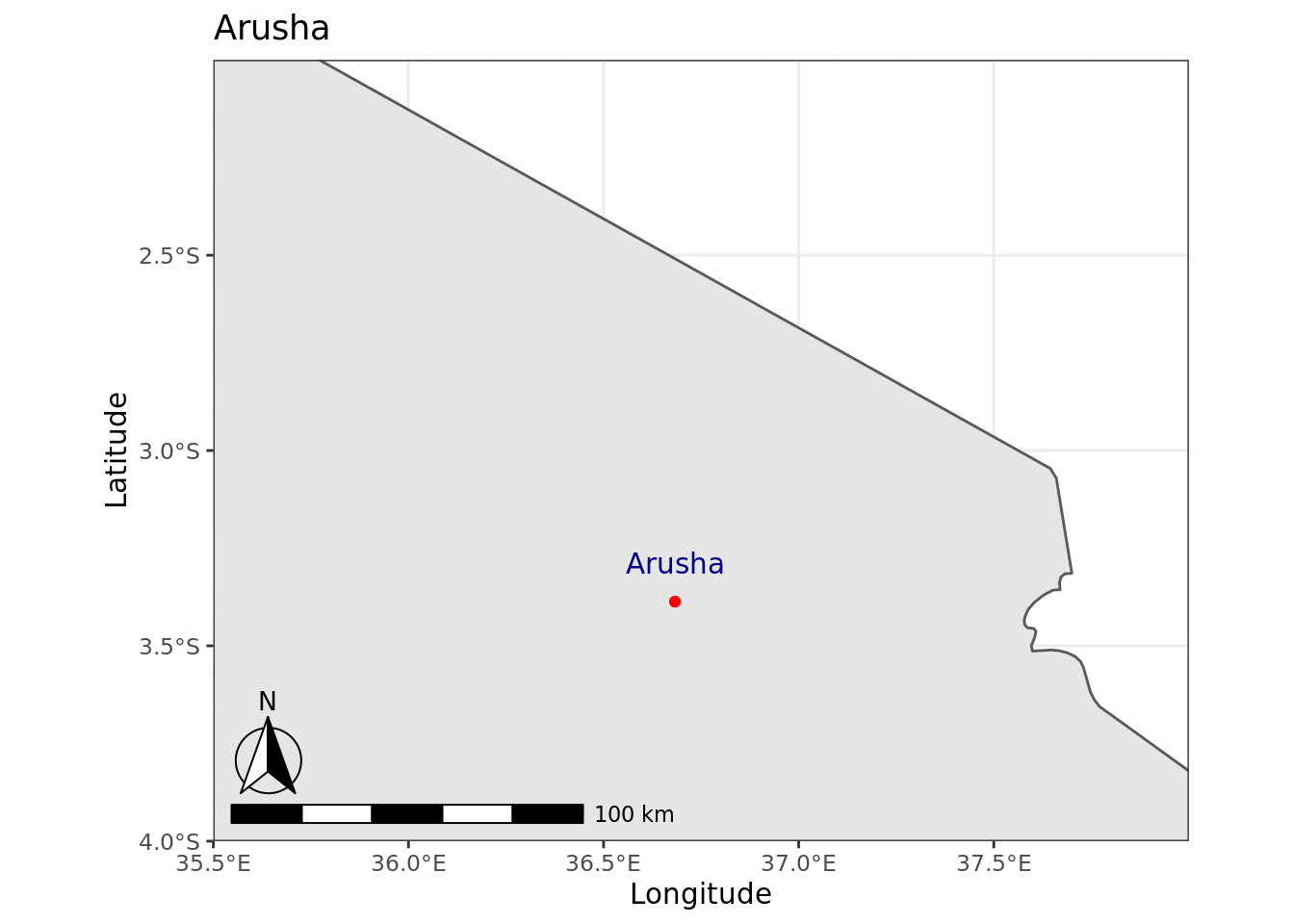## 3.10 Static maps using the `tmap` package

The `tmap` package is designed to make building thematic maps easy. It is also integrated nicely with `ggplot2`. After installing `tmap`, it takes just a few lines of code to make a global map of the distribution of the Human Poverty Index (HPI) as shown below.

``````library(tmap)
data("World")

tm_shape(World) +
tm_polygons("HPI")``````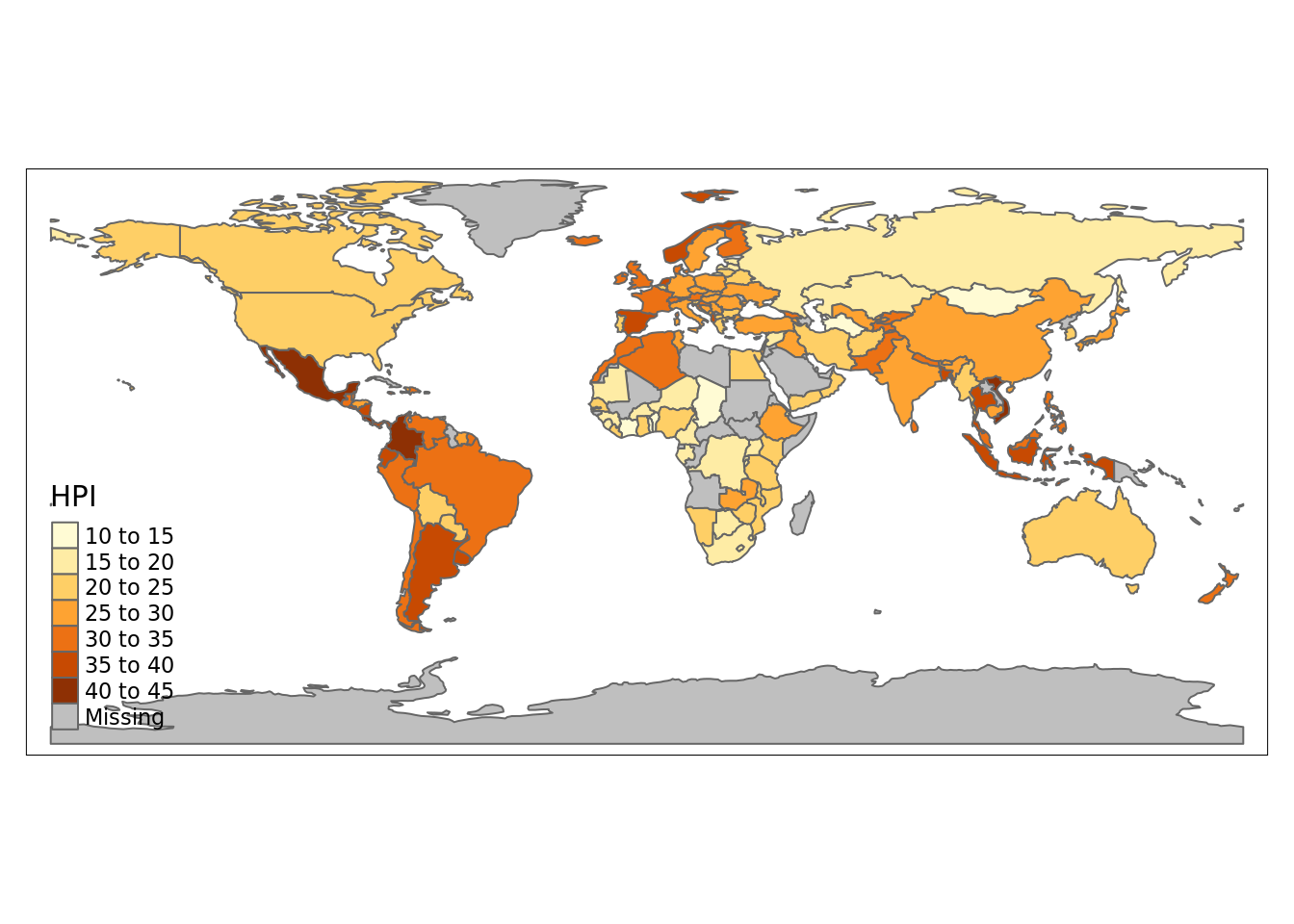The object `World` is a spatial object of class `sf` from the sf package; it is a data.frame with a special column that contains a geometry for each row, in this case polygons representing each nation. In order to plot it in `tmap`, you first need to specify it with `tm_shape`. Layers can be added with the `+` operator, in this case `tm_polygons`. There are many layer functions in `tmap`, which can easily be found in the documentation by their `tm_` prefix. See also `?'tmap-element'`.

### 3.10.1 Thematic Map of Life Expectancy among African Nations

`tmap` is great for generating thematic maps, and there are many ways that you can customize the layout and appearances of your maps, using the function `tm_layout`. Below, we plot life expectancy at birth across different African nations. We also add a title and a compass.

``````africa =  World[World\$continent == "Africa",]
tm_shape(africa) +
tm_layout(title = "Life Expectancy in Africa",
inner.margins=c(.15,.1,.2,.1)) +
tm_polygons(col = "life_exp", palette = "BuGn") +
tm_compass(type = "8star", position = c("right", "bottom"))``````